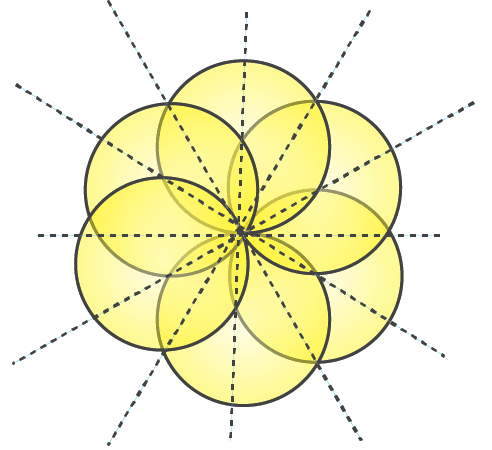# RD Sharma Solutions For Class 7 Maths Chapter 18 Symmetry Exercise 18.1

To make learning easy for students RD Sharma Solutions for Class 7 Maths Chapter 18 Symmetry Exercise 18.1 are provided here. Our experts have formulated textbook solutions in a clear manner to help students prepare for their board exams and come out with flying colours in their examinations. RD Sharma Solutions for Class 7 provides solutions for all chapters covered in the textbook. This exercise deals with the lines of symmetry. Some of the important topics of this exercise are listed below.

• Lines of symmetry
• Lines of symmetry of a line
• Lines of symmetry of a line segment
• Lines of symmetry of an angle
• Lines of symmetry of an isosceles triangle
• Lines of symmetry of a parallelogram
• Lines of symmetry of a rhombus
• Lines of symmetry of a rectangle
• Lines of symmetry of an isosceles trapezium
• Lines of symmetry of a kite
• Lines of symmetry of some regular polygons

## Download the PDF of RD Sharma Solutions For Class 7 Maths Chapter 18 – Symmetry Exercise 18.1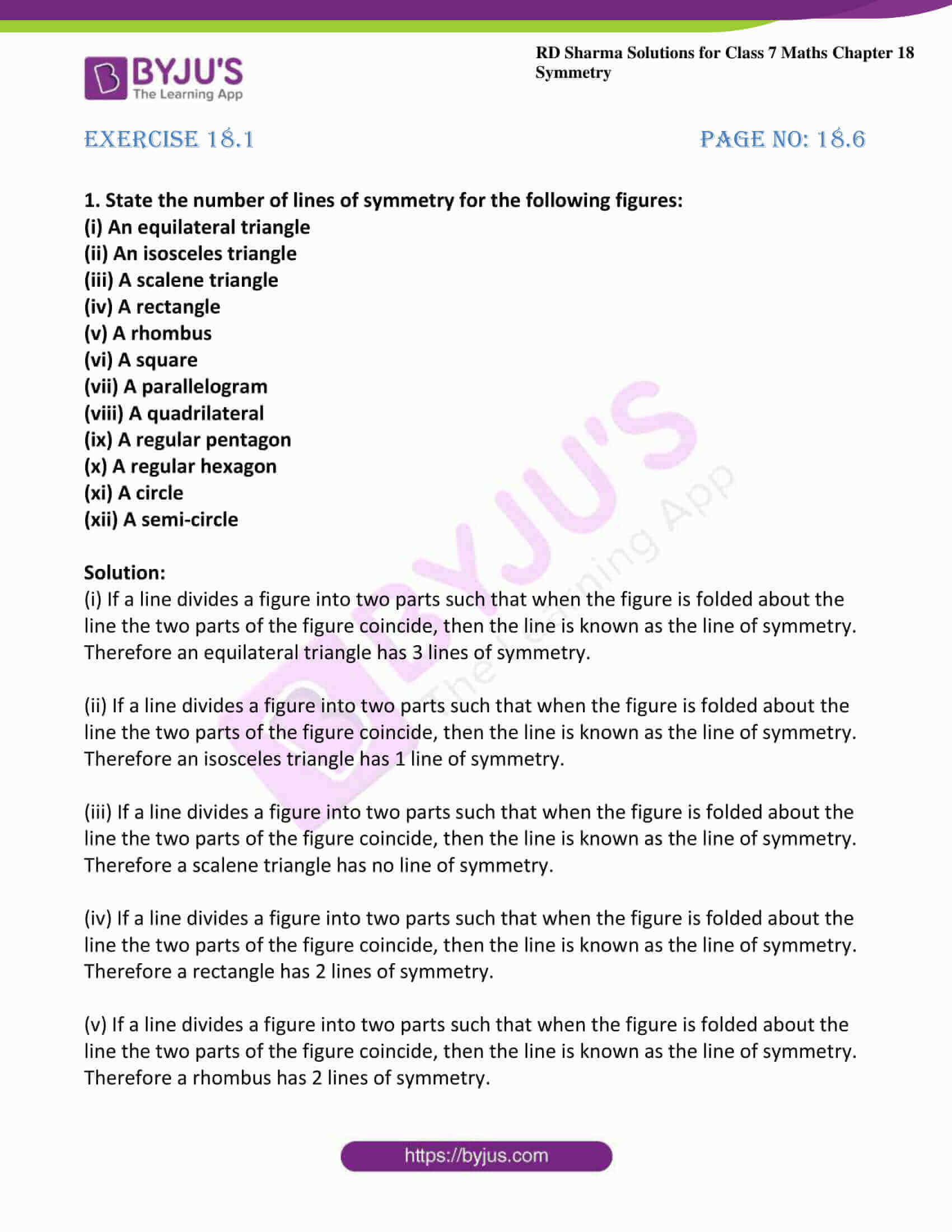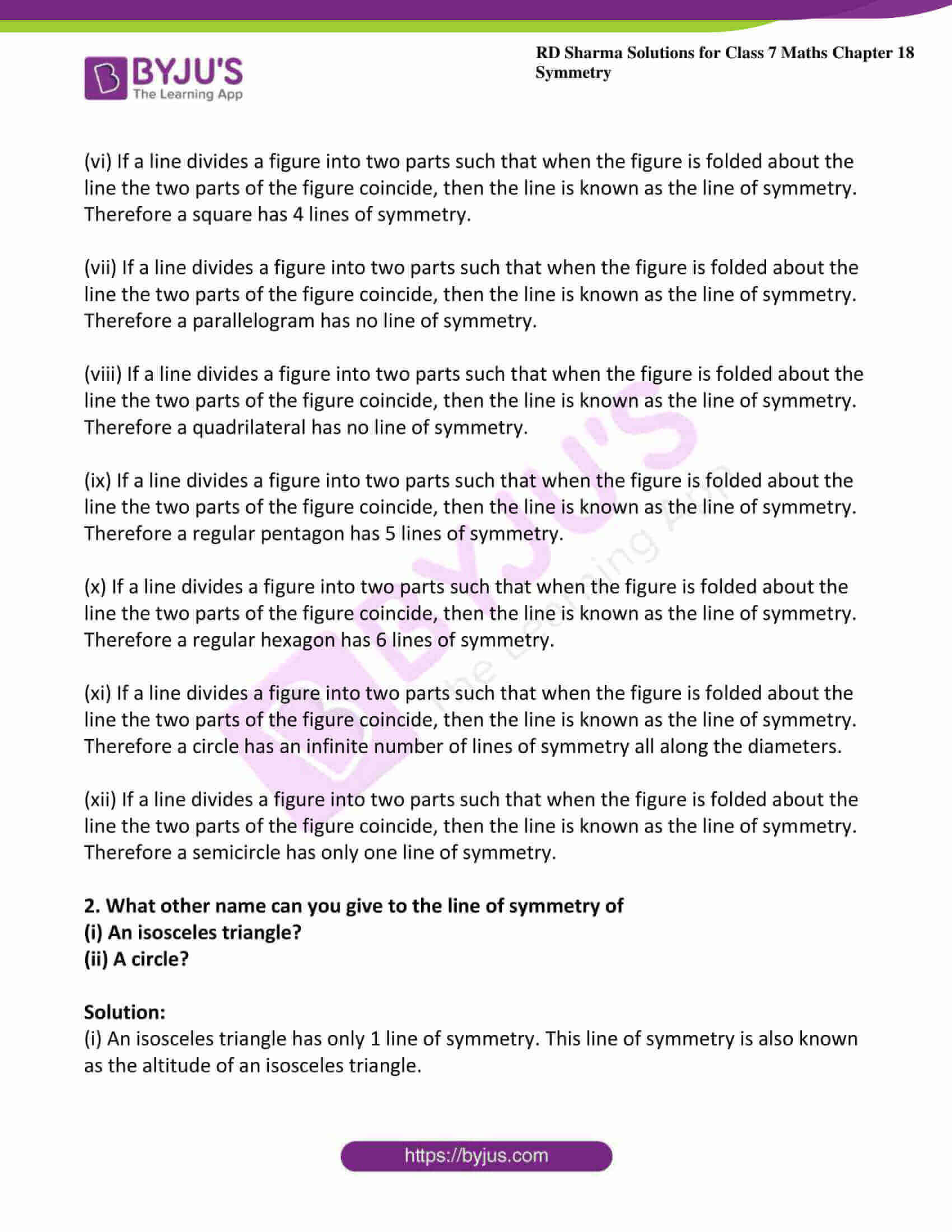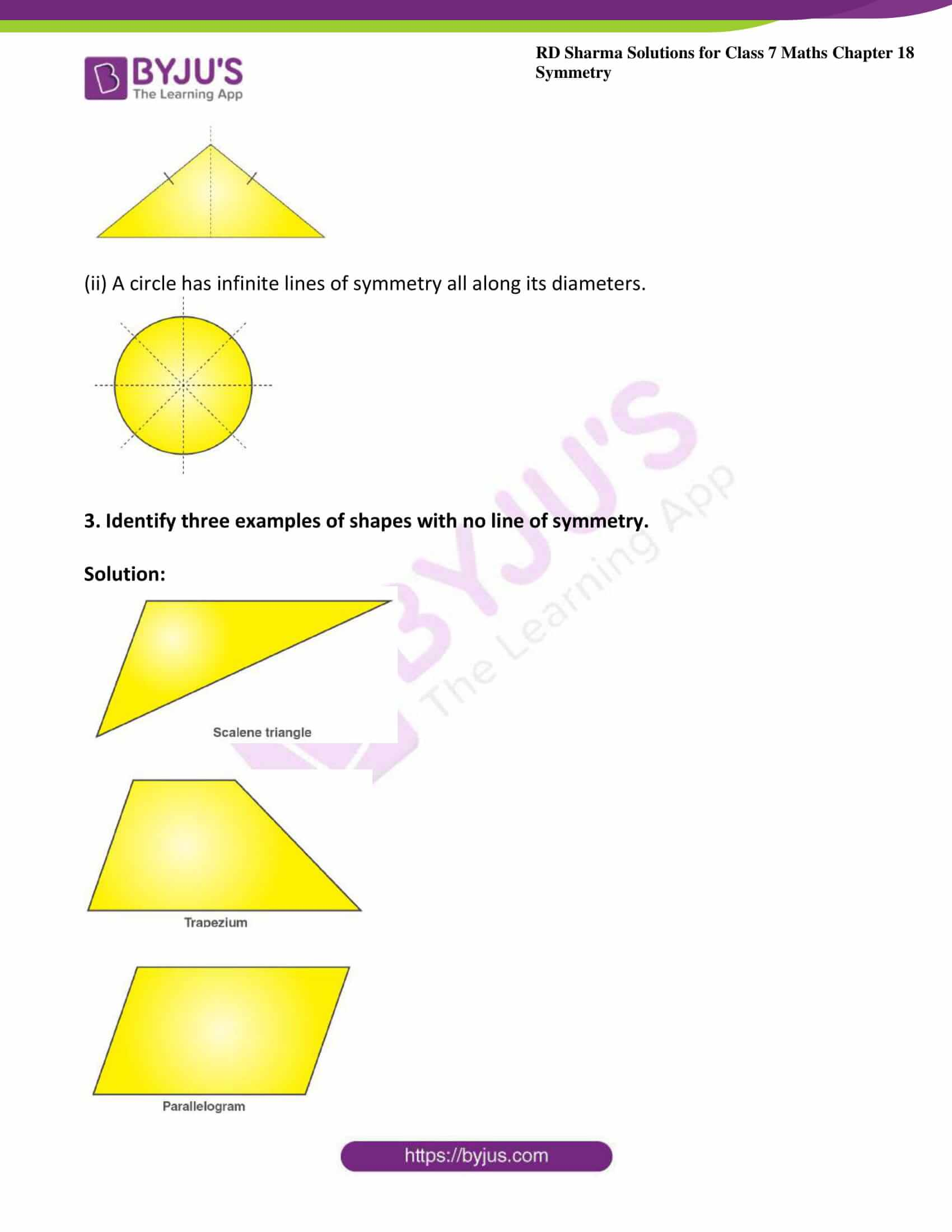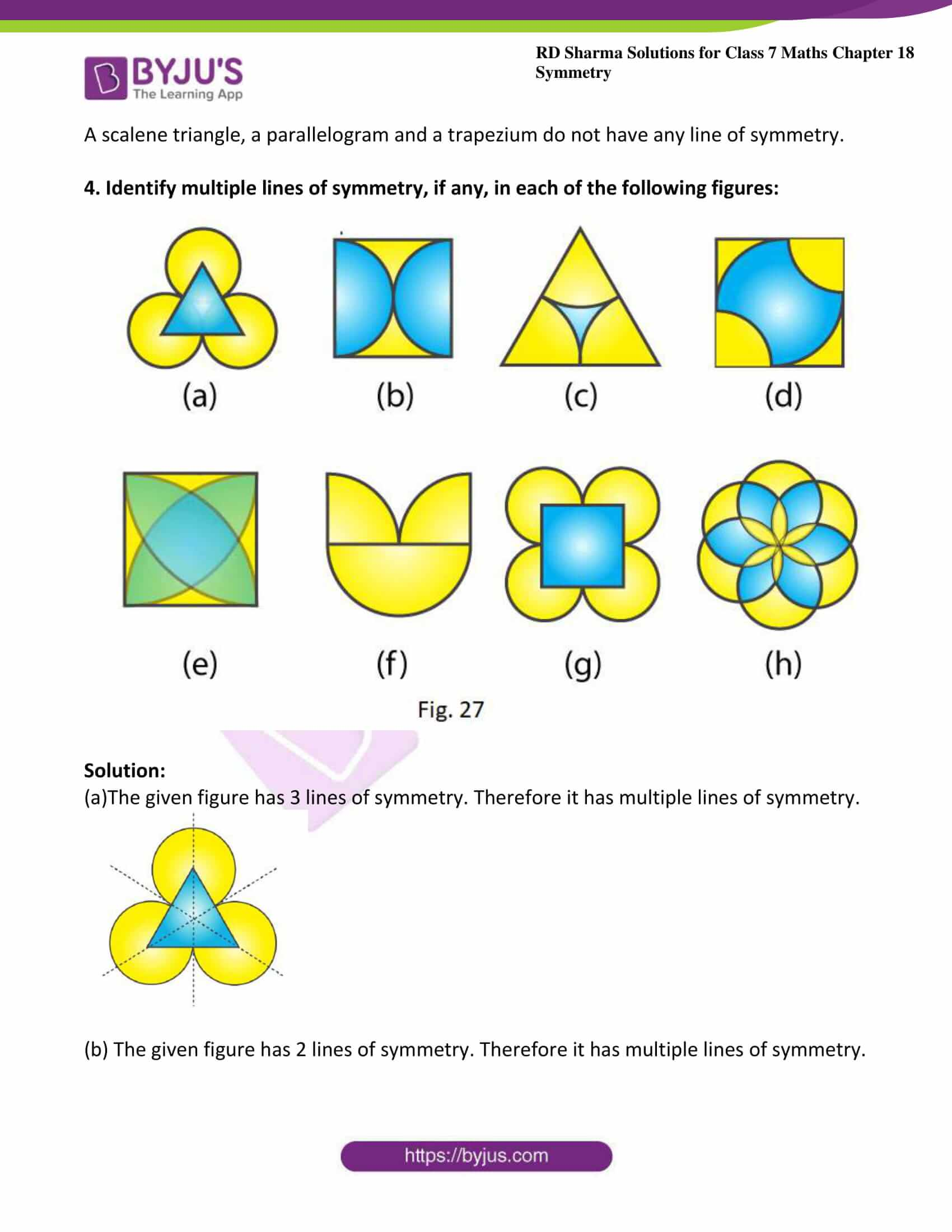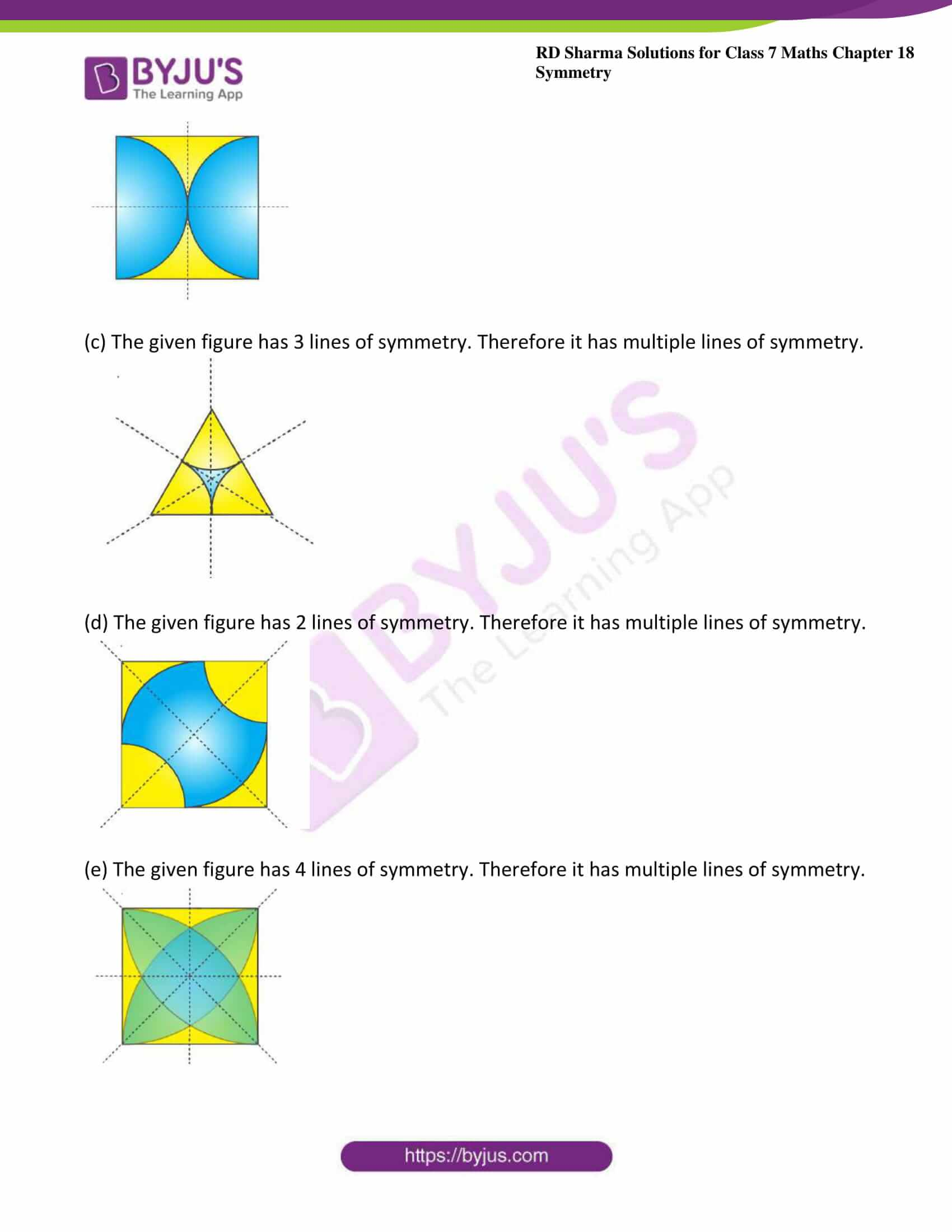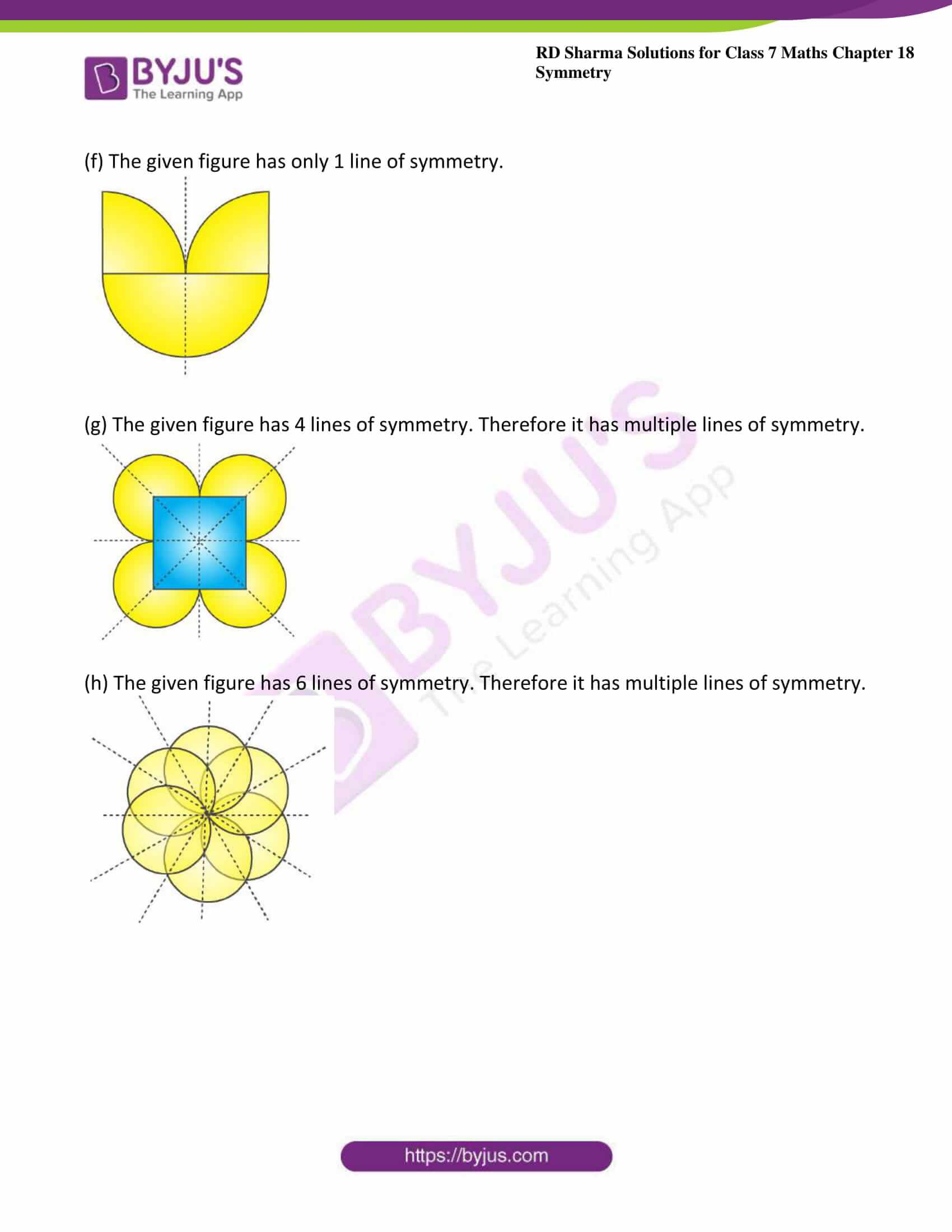### Access answers to Maths RD Sharma Solutions For Class 7 Chapter 18 – Symmetry Exercise 18.1

Exercise 18.1 Page No: 18.6

1. State the number of lines of symmetry for the following figures:

(i) An equilateral triangle

(ii) An isosceles triangle

(iii) A scalene triangle

(iv) A rectangle

(v) A rhombus

(vi) A square

(vii) A parallelogram

(ix) A regular pentagon

(x) A regular hexagon

(xi) A circle

(xii) A semi-circle

Solution:

(i) If a line divides a figure into two parts such that when the figure is folded about the line the two parts of the figure coincide, then the line is known as the line of symmetry.

Therefore an equilateral triangle has 3 lines of symmetry.

(ii) If a line divides a figure into two parts such that when the figure is folded about the line the two parts of the figure coincide, then the line is known as the line of symmetry.

Therefore an isosceles triangle has 1 line of symmetry.

(iii) If a line divides a figure into two parts such that when the figure is folded about the line the two parts of the figure coincide, then the line is known as the line of symmetry.

Therefore a scalene triangle has no line of symmetry.

(iv) If a line divides a figure into two parts such that when the figure is folded about the line the two parts of the figure coincide, then the line is known as the line of symmetry.

Therefore a rectangle has 2 lines of symmetry.

(v) If a line divides a figure into two parts such that when the figure is folded about the line the two parts of the figure coincide, then the line is known as the line of symmetry.

Therefore a rhombus has 2 lines of symmetry.

(vi) If a line divides a figure into two parts such that when the figure is folded about the line the two parts of the figure coincide, then the line is known as the line of symmetry.

Therefore a square has 4 lines of symmetry.

(vii) If a line divides a figure into two parts such that when the figure is folded about the line the two parts of the figure coincide, then the line is known as the line of symmetry.

Therefore a parallelogram has no line of symmetry.

(viii) If a line divides a figure into two parts such that when the figure is folded about the line the two parts of the figure coincide, then the line is known as the line of symmetry.

Therefore a quadrilateral has no line of symmetry.

(ix) If a line divides a figure into two parts such that when the figure is folded about the line the two parts of the figure coincide, then the line is known as the line of symmetry.

Therefore a regular pentagon has 5 lines of symmetry.

(x) If a line divides a figure into two parts such that when the figure is folded about the line the two parts of the figure coincide, then the line is known as the line of symmetry.

Therefore a regular hexagon has 6 lines of symmetry.

(xi) If a line divides a figure into two parts such that when the figure is folded about the line the two parts of the figure coincide, then the line is known as the line of symmetry.

Therefore a circle has an infinite number of lines of symmetry all along the diameters.

(xii) If a line divides a figure into two parts such that when the figure is folded about the line the two parts of the figure coincide, then the line is known as the line of symmetry.

Therefore a semicircle has only one line of symmetry.

2. What other name can you give to the line of symmetry of

(i) An isosceles triangle?

(ii) A circle?

Solution:

(i) An isosceles triangle has only 1 line of symmetry. This line of symmetry is also known as the altitude of an isosceles triangle.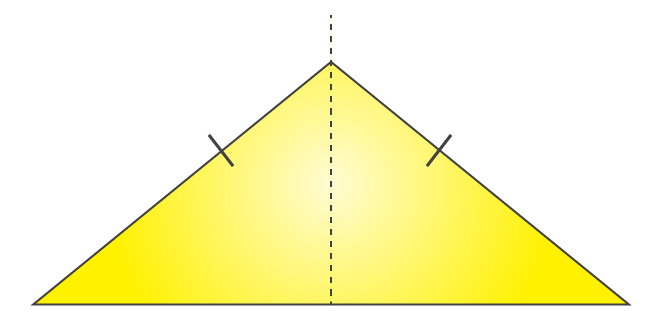(ii) A circle has infinite lines of symmetry all along its diameters.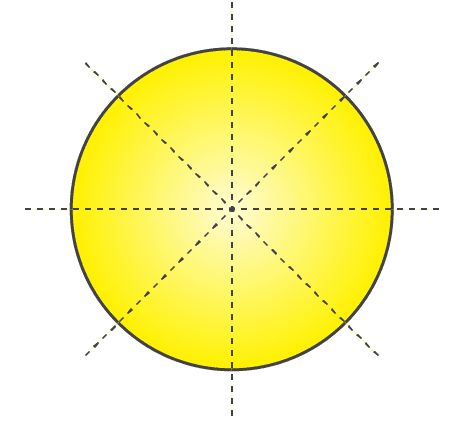3. Identify three examples of shapes with no line of symmetry.

Solution: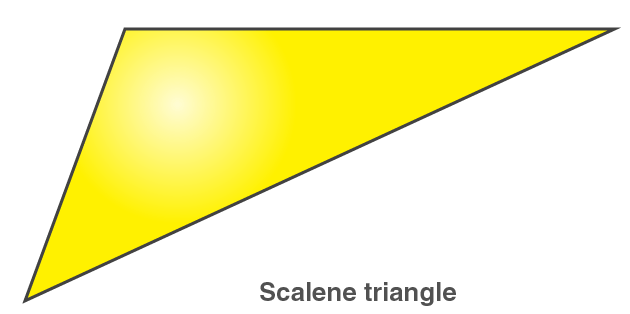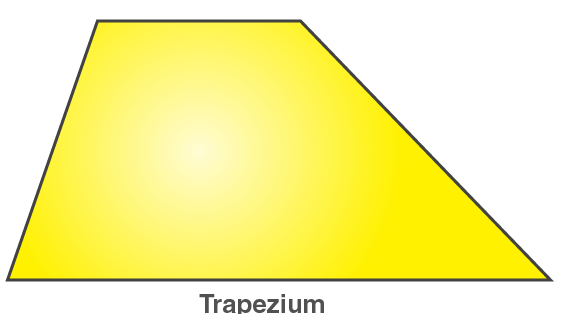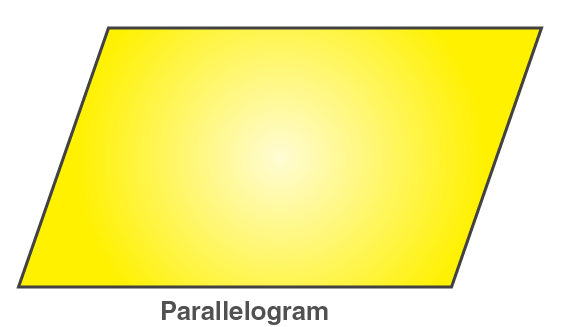A scalene triangle, a parallelogram and a trapezium do not have any line of symmetry.

4. Identify multiple lines of symmetry, if any, in each of the following figures: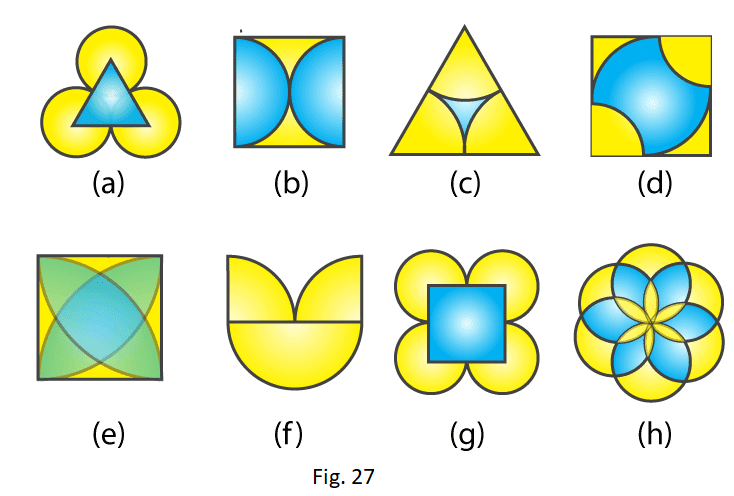Solution:

(a)The given figure has 3 lines of symmetry. Therefore it has multiple lines of symmetry.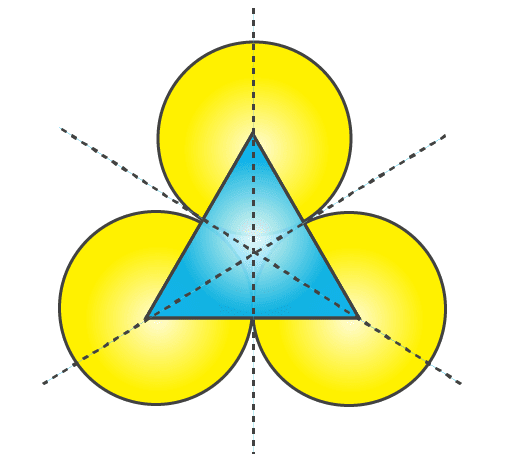(b) The given figure has 2 lines of symmetry. Therefore it has multiple lines of symmetry.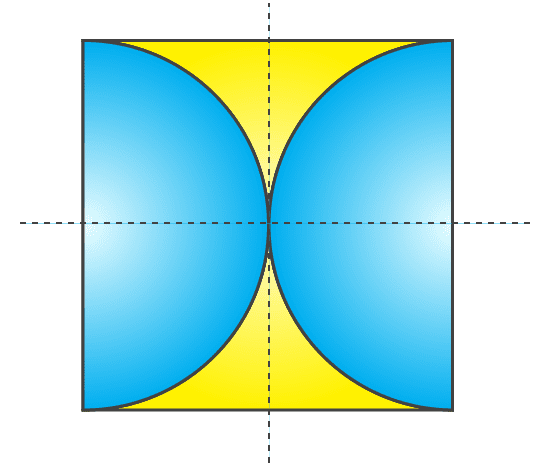(c) The given figure has 3 lines of symmetry. Therefore it has multiple lines of symmetry.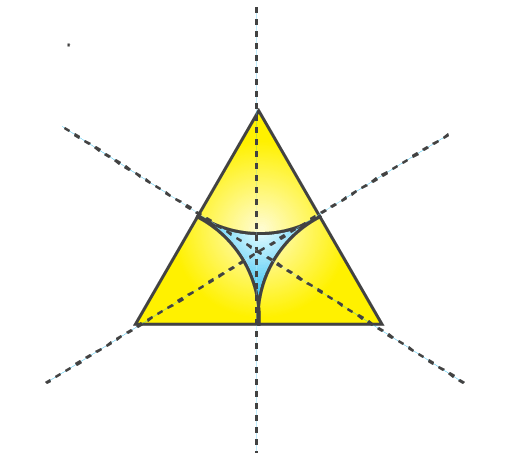(d) The given figure has 2 lines of symmetry. Therefore it has multiple lines of symmetry.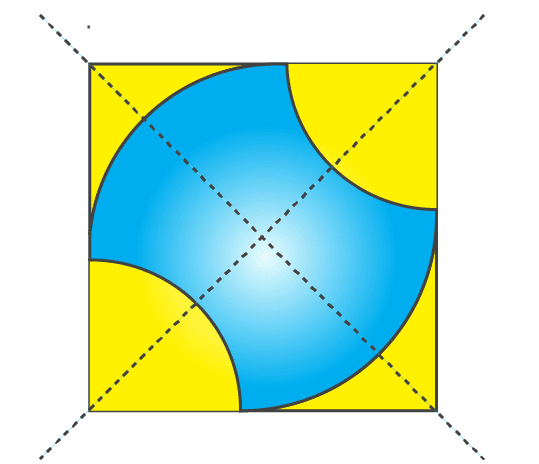(e) The given figure has 4 lines of symmetry. Therefore it has multiple lines of symmetry.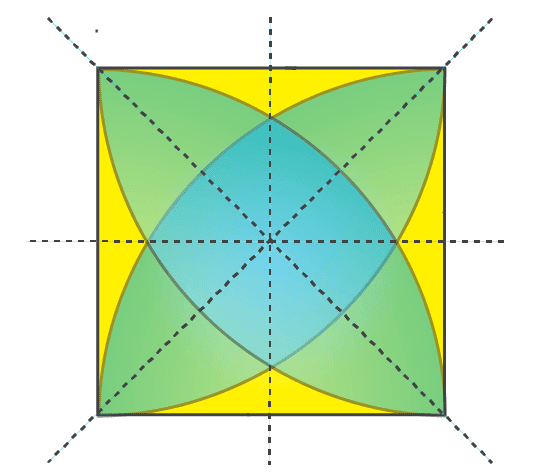(f) The given figure has only 1 line of symmetry.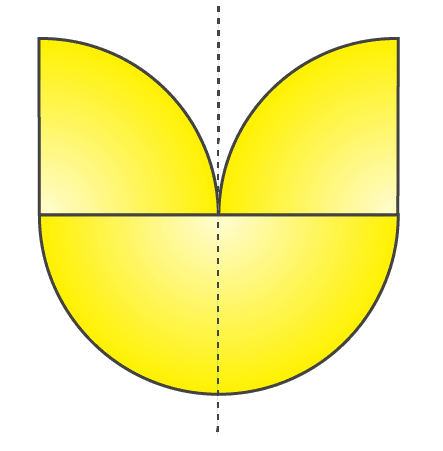(g) The given figure has 4 lines of symmetry. Therefore it has multiple lines of symmetry.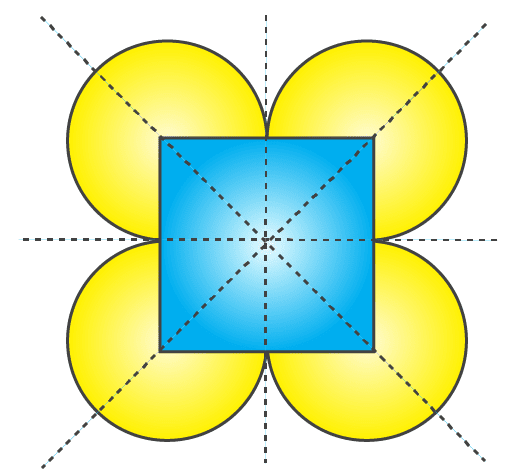(h) The given figure has 6 lines of symmetry. Therefore it has multiple lines of symmetry.